Latest Banking jobs   »   Reasoning Ability Quiz For IBPS PO...

# Reasoning Ability Quiz For IBPS PO Prelims 2021- 8th December

Directions (1-3): Study the following information carefully and answer the given questions.
There are seven members in a family i.e. A, B, C, D, E, F and G. Among them only two are married couple and only three are males. A who is unmarried, and F are siblings. B’s husband has two children. D is father of F. G is niece of A, who is not a male member. B is mother of C, who is unmarried. E is not married to F or G.

Q1. Who among the following is Aunt of C?
(a) D
(b) E
(c) G
(d) A
(e) None of these

Q2. Who among the following is grandmother of G?
(a) E
(b) F
(c) B
(d) C
(e) None of these

Q3. How B related to A?
(a) Sister
(b) Aunt
(c) Mother
(d) Sister-in-law
(e) Brother

Directions (4-5): Read the following information carefully and answer the questions given below.
A # B means ‘A is son of B’
A \$ B means ‘A is wife of B’
A * B means ‘A is sister of B’
A @ B means ‘A is brother of B’
A & B means ‘A is father of B’

Q4. If ‘A # B \$ C @ D & E’, which of the following is true?
(a) E is the sister of C
(b) C is an aunt of A
(c) D is the brother-in-law of B
(d) A is the son of D
(e) None is true

Q5. Which of the following shows that R is the daughter-in-law of S?
(a) Q & R * T @ P # U * S
(b) Q \$ R & T @ P * S & U
(c) R & Q * T @ P \$ U & S
(d) Q & R \$ T @ P # S & U
(e) None of these

Directions (6-8): Study the following information carefully to answer the following question:
There are eight members in the family having three generations. There are only three married couple. A is mother of D. G is son in law of B. H is nephew of D. C has only one son. F is granddaughter of C. E is mother of F. D is unmarried.

Q6. How C is related to D?
(a) Father
(b) Mother
(c) Son
(d) Son in law
(e) Brother

Q7. Who among the following is son in law of E?
(a) A
(b) C
(c) H
(d) G
(e) None of these

Q8. Who among the following is niece of D?
(a) C
(b) E
(c) H
(d) F
(e) G

Directions (9-10): Each of these questions is based on the following information:
(i) P % Q means P is the father of B.
(ii) P @ Q means P is the sister of B.
(iii) P \$ Q means P is the brother of B.
(iv) P * Q means P is the son of B.

Q9. In the expression ‘S \$ R % Q @ Y * M’, how is M related to S?
(a) Aunt
(b) Mother
(c) Sister
(d) Sister in law
(e) None of these

Q10. In the expression N @ M * A \$ S % Z, how is S related to N?
(a) Aunt
(b) Uncle
(c) Sister
(d) Sister in law
(e) None of these

Directions (11-13): Study the following information carefully and answer the given questions:
M, P, Q, R, S, T and W are seven persons of three generation living in the same house. There are two married couple in the house. M is the only daughter of P, who is the paternal grandfather of T. R is the son of Q. T is the daughter of S. R is the father of W. T is sister of W.

Q11. How is M related to S?
(a) Mother in-law
(b) Sister in-law
(c) Father in-law
(d) Sister
(e) Can’t determined

Q12. How is W related to R?
(a) Son
(b) Father
(c) Daughter
(d) Can’t determined
(e) Mother

Q13. Which of the following is the pair of “husband-wife”?
(a) P, W
(b) T, M
(c) M, S
(d) Q, M
(e) R, S

Directions (14-15): Study the following information carefully and answer the questions given below:
There are Seven Members A, B, C, D, E, F and L in a family. There are two married couples and only three generations in the family. F is grandmother of B, who is niece of D. F has one son and one daughter. C is brother-in-law of D. L is grandfather of E. B has one sibling.

Q14. How is F related to mother of B?
(a) sister
(b) grandmother
(c) daughter
(d) mother
(e) wife

Q15. How is C related to child of A?
(a) Grandfather
(b) father
(c) Uncle
(d) Brother
(e) Cousin

Solutions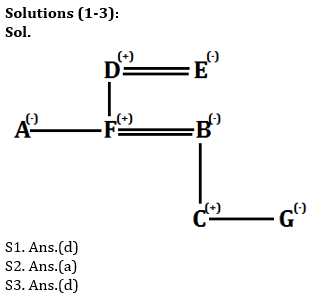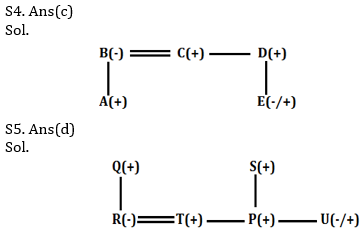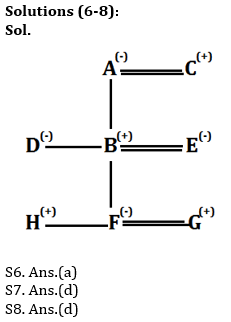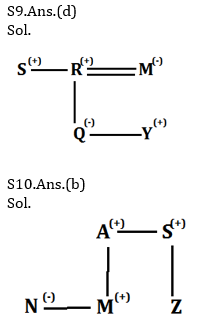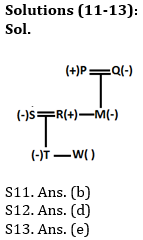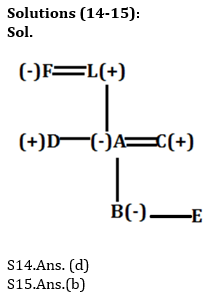Click Here to Register for Bank Exams 2021 Preparation Material#### Congratulations!Download Hindu Review of October 2021: Free PDF